telescopeѲptics.net          ▪▪▪▪                                             CONTENTS

# 9.3. DESIGNING DOUBLET ACHROMAT

Designing doublet lens objective is quite complex procedure. The reason is obvious: there is four optical surfaces, whose synergistic effect needs to correct both monochromatic and chromatic aberrations. Follows one possible solution. The goal here is a coma-free air-spaced doublet achromat (contact type), also corrected for spherical aberration. This doublet type is known as Fraunhofer. Correction of astigmatism will not be required, since it is independent of radii for given lens power, and typically low (unless a doublet is of large relative aperture, in which case it is the secondary spectrum that is more detrimental optically).

Starting with the requirement for minimized chromatism, the individual focal lengths of the two lens elements need to relate as the reciprocal of their Abbe numbers (Eq. 42):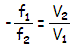with f1, f2 being the focal length of the front and rear element, respectively (commonly used Abbe number is for the d-line - Vd - but using Ve does not have appreciably different effect, because its values for both glasses change nearly proportionally). The individual focal lengths relate to the doublet focal length f as f=f1 f2/(f 1+f2). From Eq. 1.2, lens focal length in terms of its refractive index n and front and rear radius R1 and R2, respectively, is f=R1R2/(n-1)(R 2-R1), and focal length of two combined thin lenses is given with Eq. 1.2.1/1.2.2.

Note that f2 is numerically negative.

In terms of the system focal length, the individual lens focal lengths are (Eq. 43):(a)

While formally any combination of Abbe numbers could be used, the practical requirement is that the two Abbe numbers are significantly different in their nominal values, roughly in V1:V2~2:1 ratio (the inverse ratio - Steinheil type doublet - requires significantly more strongly curved lenses). The lower ratio, the more strongly curved lenses have to be.

As Eq. 46 indicates, the amount of secondary spectrum varies - possibly significantly - with the relative partial dispersion of the two glass types. The usual choice are n~1.52 borosilicate crown and n~1.62 flint (for instance, BK7 and F2), resulting in near-minimum secondary spectrum possible with common glasses.

Graph below (source: ATMOS) shows relative partial dispersion PF,e for optical glasses produced by major manufacturers. The smaller PF,e differential, and the greater V differential between two doublet glasses, the lower its secondary spectrum. A doublet made from BK7 crown and F3 flint will have secondary spectrum close to the minimum possible with common glasses (~1/2000f); if F3 is replaced with, say, KZFS1 (several times more expensive), secondary spectrum will be further reduced by nearly 40%. In effect, the amount of secondary spectrum produced by a doublet is proportional to the ratio of the height vs. base of the right-angle triangle whose hypotenuse connects the two glasses' relative partial dispersions (gray triangle for BK7 and F3). Specifically, it is given by the ratio of differential in PF,e vs. differential in V (Eq. 46).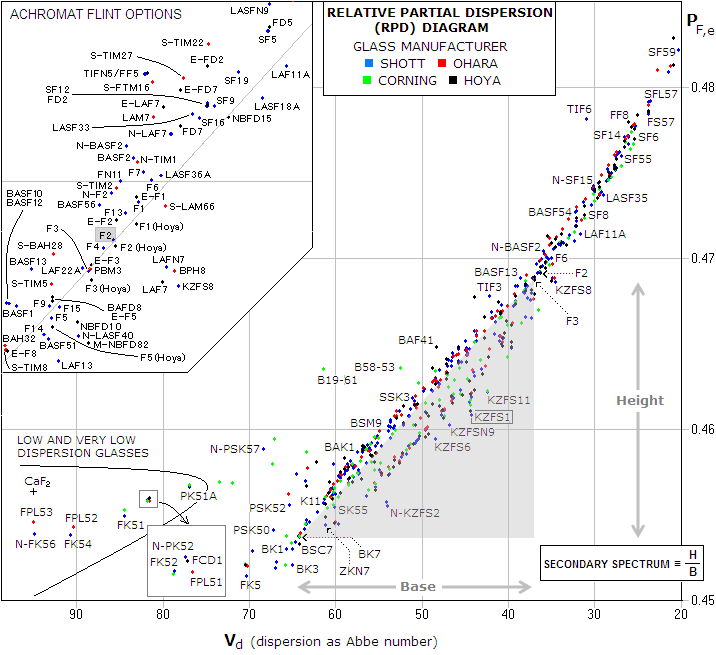FIGURE 150: Secondary spectrum (Δf) in the refracting objective is proportional to the ratio between the height (H) and the base (B) of the triangle formed around the line connecting two glasses, Δf=(H/B)f, with f being the focal length. Numerically, H equals the relative partial dispersion differential, and B the Abbe number differential between the two glasses. Obviously, the greater slope of the line connecting two glasses, the larger secondary spectrum; near-horizontal connecting line indicates practically non-existent secondary spectrum. Flint glasses around the typical F2 Schott match for BK7 are numerous (top left), but would not appreciably affect the secondary spectrum. Those above the gray diagonal line, connecting F2 with BK7, have slightly larger slope, thus slightly greater secondary spectrum; those below the line have it slightly smaller.

Obviously, it is possible to select two glasses that would practically cancel secondary spectrum. However, such combination in a doublet often requires significantly more strongly curved inner two surfaces, due to typically smaller difference between dispersive powers of the two elements (a consequence of the limited inventory of existing glasses). This in turn may induce significant higher-order spherical aberration.

In principle, any two glasses will have very similar degree of secondary spectrum correction, regardless of the objective type (i.e. doublet or triplet). The main advantage of triplet objective is that the third element allows for significantly more relaxed curvatures, which not only helps control higher-order spherical and spherochromatism, but also makes fabrication/alignment tolerances much more relaxed (this is very important in apochromatic objectives, especially at faster focal ratios).

In order to keep design calculations relatively simple, following design scheme is limited to the common glasses, although it should also be sufficiently accurate, at lest as a preliminary design, for semi-apo and smaller long-focus apochromatic objectives.

Once the glass combination is determined, both Abbe numbers and refractive indici - V1 and V2, n1 and n2, for the front and rear lens, respectively - are known.

The next step is choosing correction type, that is, which two separate wavelengths will be brought to a common focus. This in turn determines the lens bending parameter k in terms of refractive indici for the two distant wavelengths to be brought to a common focus. If we choose to bring together F and C lines, the bending parameter ratio is given by: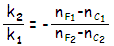(Eq. 44), with k=(1/R1)-(1/R2), R1,2 being the front and rear lens radius of curvature, respectively, and nF1, nC1 and nF2, nC2 refractive indici of the selected distant wavelengths for the front and rear lens, respectively (F and C spectral lines are the usual choice; better overall alternative is to bring together d and F lines, which would result in slightly different lens bending ratio).

With the front and rear surface radius of each lens element being related in terms of the lens shape factor q=(R2+R1)/(R2-R1) as R2=(q+1)R1/(q-1), substituting  R2=(q1+1)R1/(q1-1) and R4=(q2+1)R3/(q2-1) in k2/k1 gives: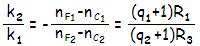(b)

with q1 and q2 being the lens shape factor for the front and rear lens, respectively. This determines the relation between the first and third radius needed to bring selected wavelengths to a common focus.

The lens shape factor q is determined according to the requirement for corrected monochromatic aberrations. For corrected coma, the sum of aberration coefficients c1 and c2 for the front and rear lens, respectively, must be zero. Coma aberration coefficient for thin lens is: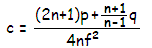(c)

with n being the refractive index, p the position factor, and f the lens focal length.

Position factor p=1-(2f/I), with f being the lens focal length, and I the lens-to-image separation, is given by p1=1-(2 f1/I 1)=-1 for the front lens (since image distance I1 equals the focal length f1 for distant objects), and with f2=(V 2-V1)f/V 2 from Eq. 43, position factor for the rear lens p2=1-(2f2 /I2)=(2V1 -V2)/V2 (since I2=f).

Setting c1+c2=0 (i.e. c1=-c2) and substituting for the refractive indici and position factors, allows to extract a ratio between shape factors for the front and rear lens needed to cancel coma as q2=xq1.

Next step is setting the aberration coefficients for spherical aberration to zero and, after substituting xq1 for q2 in the rear lens aberration coefficient expression, consolidating the expression into quadratic equation and solve it for q1. Aberration coefficients  s1 and s2 for primary spherical aberration for each lens element are obtained from the general coefficient relation (Eq. 49):(d)

after substituting the appropriate values for n, p and f for the specific lens element. The index n is that for the chosen optimized wavelength, usually 546nm e-line.

The peak aberration coefficient value for primary spherical aberration is proportional to the fourth power of the effective lens aperture. Due to the positive front lens, the effective aperture is slightly smaller for the rear lens, and so is its peak aberration coefficient, approximately by a factor of [1-t/f1]4, with t being the axial lens separation and f1 the front lens f.l.. To account for this, the value of rear lens aberration coefficient s (i.e. s2) obtained from Eq. (d) should be reduced according to this factor. For typical medium-to-long focus achromats this value is negligibly small; for instance, in a 100mm f/12 achromat with f1~520mm and t~1mm, the actual coefficient value for the rear lens would have been only 0.8% smaller than what the unadjusted expression implies.

Obviously, the calculation is extensive, but fairly straightforward, simplifying greatly after substituting for n. Once the sum of two coefficients is consolidated to s1+s2=aq12+bq1+c=0 quadratic form, the solution for q1 is given by q=[-b±(b2-4ac)1/2]/2a. Of the two solutions for the shape factor q1, the one to use is numerically negative, usually roughly midway between -0.5 and zero (for biconvex lens with the rear surface more strongly curved). For the rear lens, the shape factor q2=xq1 is numerically positive, around ~1.6, being a negative meniscus with the first surface strongly curved, and weak rear surface curvature.

After the shape factor q is determined for both lens elements, the appropriate surface radii are obtained from: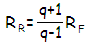with RR, RF being the rear and front surface radius of the lens element, respectively. This defines relation of the front and rear radius for the two lens elements; it now needs to be combined with the relation between the front lens radii (q1+1)R1/(q2+1)R3=-(nF1-nC1)/(nF2-nC2) needed to bring the two selected wavelengths to a common focus.

All these radii relations are relative to each other. Based on the R1 to R3 ratio, the R2 and R4 are determined relative to R1 and R3, respectively. What is left to do is to determine relative relation of one of those radii with respect to doublet's focal length, so that specific value for that radius, and for the other three can be obtained. With the focal length of a thin lens f=R1R 2/(n-1)(R 2-R 1), substituting for f1 and f2 in the relation for doublet's focal length f=f1f2/(f1+f2) gives doublet focal length defined in terms of lens indici and the four radii as: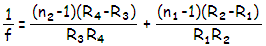Next step is substituting R2=(q1+1)R1/(q1-1) and R4=(q2+1)R3/(q2-1), which defines (1/f) in terms of indici and R1 and R3; after substituting R3=-(n F2-nC2 )(q1+1)R1 /(nF1-n C1)(q2+1), from the relation needed to bring the two selected wavelengths to a common focus, the doublet focal length is defined in terms of the optimized wavelength indici n1 (front lens) and n2 (rear lens), the front lens shape factor q1 and first radius R1 as (1/f)=2[(n1 -1)-(n2-1)(n F1-nC1)/(n F2-nC2)]/(q 1+1)R1. This  defines R1 in terms of the doublet focal length f as: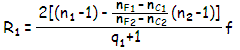To summarize the steps, the design procedure unfolds in the following order:

1 - decide the focal length f of the doublet, and calculate needed f.l. for each lens for chosen glasses from Eq. (a)

2 - decide the type of correction, i.e. which two colors will be brought to a common focus; for the standard F-C correction on, us (nF1-nC1) and (nF2-nC2), for F-d correction (shifted toward the blue end) use (nF1-nd1)/(nF2-nd2), and for e-C correction mode, shifted toward the red, use (ne1-nC1)/(ne2-nC2).

3 - set the sum of coma coefficients for the two elements to zero and extract the ratio of shape factors q2=xq1 needed to correct coma, as described with Eq. (c) and following relations

4 - substitute xq1 for q2 in the rear lens aberration coefficient for spherical aberration and set the sum of the coefficients for the two lenses, based on Eq. (d), to zero, in order to get quadratic equation of the form aq12+bq1+c=0 with two solutions for the front lens shape factor, q1,2=[-b±(b2-4ac)1/2]/2a; use numerically negative solution q1=y, which determines the rear lens shape factor as q2=xy (taking the positive solution is valid too, but it results in a harder to make aplanatic doublet, consisting of a positive and negative meniscus - Gauss doublet).

5 - calculate the radii from: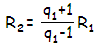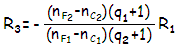Note that R1 and R3 here are determined for C and F lines focusing together.

EXAMPLE: Designing a 100mm f/10 Fraunhofer doublet. Taking BK7 crown and F2 flint (Schott catalogue) for the front and rear lens, respectively, gives the following glass parameters:

 PARAMETER BK7 F2 nC nC1=1.514322 nC2=1.615032 nd nd1=1.516800 nd2=1.620040 ne ne1=1.518722 ne2=1.624080 nF nF1=1.522376 nF2=1.632081 Abbe number (Ve) V1=64.4 V2=36.6

1 - With f=1,000mm, V1=64.4 and V2=36.6, the needed focal length for the front lens, from Eq (a), equals
f
1=(V1-V2)f/V1=432mm, and for the rear lens f2=(V2-V1)f/V2=-760mm.

2 - Next step, determining lens shapes that will cancel coma, requires a sum of coma coefficients for the two elements equal to zero, i.e. c1+c2=0. With the position factor for the front lens p1=-1 for object at infinity, and
p
2=(2V1-V2)/V2=2.52, from Eq. (c), the corresponding coma coefficients are

c1={(2ne1+1)p1+[(ne1+1)/(ne1-1)]q1}/4ne1f12=0.8q1-0.665 and

c2={(2ne2+1)p2+[(ne2+1)/(ne2-1)]q2}/4ne2f22=0.209q2+0.532,

where q1 and q2 are the shape factors for the front and rear element, respectively. Note that since we don't need the actual coefficient values, only their relationship, the calculation is simplified by setting f1=1 and f2=f2/f1. Equaling the sum of coefficients with zero gives q2=0.64-3.83q1.

3 - With the relation between shape factors needed to correct coma determined, the next and last step is to find the specific shape factors values that will correct for primary spherical aberration. These values need to be such so that a sum of aberration coefficients for the two elements is zero, i.e. s1+s2=0. Substituting corresponding n, p and q values into Eq. (d), with q2=0.64-3.83q1 and setting again f1=1 and f2=f2/f1, gives the coefficients for the two elements as:

s1=-[ne13+(ne1+2)q2+(3ne1+2)(ne1-1)2p12+4(ne12-1)p1q1]/32ne1(ne1-1)2f13 = -0.269q12+0.4q1-0.403  and

s2=-[ne23+(ne2+2)q2+(3ne2+2)(ne2-1)2p22+4(ne22-1)p2q2]/32ne2(ne2-1)2f23 = 0.489q12-0.74q1+0.302

With s1+s2=0.22q12-0.34q1-0.101=0, the quadratic equation parameters are a=0.22, b=-0.34 and c=-0.101. Substituting these values into quadratic formula q=[-b±(b2-4ac)1/2]/2a gives as the negative solution q1=-0.255. This, in turn, determines shape factor for the rear lens as q2=0.64-3.83q1=1.617.

4 - With the shape factors known, the radii can be calculated from the relations given in the summary (#5). It gives R1=0.602�=602mm, R2=-357.4mm, R3=-362.8 and R4=-1539. Note that the choice of correction type determines which refractive indici will be used for calculating R1 and R3; the choice here is the standard F-C correction, thus the indici used were nF and nC, as in the corresponding relations in the above summary.

Ray trace with standard lens thicknesses (11 and 7mm for the front and rear element, respectively) and separation (1mm) shows near perfect F-C chromatic correction and acceptable level of spherical aberration in the optimized wavelength (1/6 wave p-v, down to 1/10 wave with the separation reduced to 0.5mm).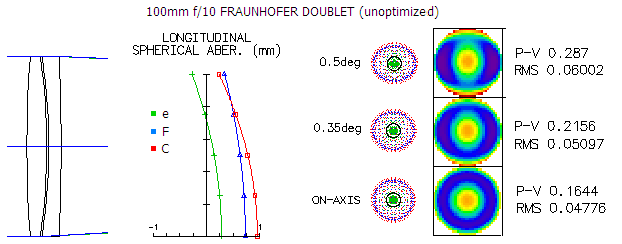More accurate (i.e. with more decimals) numbers when  calculating s1 and s2 coefficients might result in somewhat better correction of spherical aberration in the unoptimized design. The design is easily near-optimized in raytracing by reducing R3 and R1 by about 2 and 10mm, respectively.

Since the aberration contribution of the first surface is much smaller than that of the third surface, shift to a different mode of correction can achieved by a small change in R1 alone (somewhat weaker first radius shifts correction toward the red end, and slightly stronger toward the blue end, with no appreciable change in monochromatic aberrations). In this particular (unoptimized) system, R1 larger by about 40mm shifts correction mode to the red-end corrected e-C, while 30mm shorter R1 shifts it to the blue-end corrected F-d. The only appreciable effect on monochromatic aberrations is adding ~0.1 wave p-v of over-correction and under-correction, respectively (hence the system in F-d mode would require further adjustment for about 1/4 wave of under-correction, by either slightly weakening R2, or strengthening R3).

It may be convenient to have R2=-R3. Usually, R3 curvature is slightly weaker, since it induces more spherical aberration (over-correction, compensating for under-correction of the rest of surfaces) than R2. Thus equaling the inner radii requires either:
(1) larger differential in dispersive powers than in a typical doublet, since it allows for weaker inner radii, reducing their aberration differential, or
(2) wider lens separation, reducing the aberration contribution at R3 (modest separation, up to 2-3mm, usually suffices).

Finding the radii concludes preliminary design process. The doublet should be at the optimization level with respect to its optical quality. For F>10 systems such preliminary design could be quite usable without optimization, but it is always a good idea to confirm that with ray trace. With F<10 systems, sensitivity to deviations increases, and higher-order spherical aberration can become significant in the optimization procedure: ray trace is a must.

Designing with ray-tracing software

For those familiar with some of raytracing programs, designing refractor contact objective is much easier using the program's interface. Such programs usually can generate standard doublets at request. It can also be designed "manually". All that is needed is an existing objective, or its approximated radii, as those given for a few classical doublet achromats. From there, gross level of aberrations is controlled by bending surfaces while monitoring their aberration contribution.

With doublet achromat, the strongly curved two inner surfaces have by far the largest aberration contribution. Since their radii are typically similarly strong, and of opposite sign, so are their aberration contributions. Canceling of coma is done mainly by adjusting the outer radii, while keeping the combined power balance of the two elements unchanged (i.e. maintaining the overall chromatic correction). With the coma cancelled, final correction for spherical aberration is done by a minor change in one of the two inner radii, which can also be done (on a smaller scale) by changing lens' separation.

Specific steps are:

(1) selecting two glasses based on the RPD diagram

(2) bringing red and blue lines on the longitudinal aberration plot together by adjusting the inner radii; in general, strengthening the inner radii brings the red closer, while shifting blue farther away, and vice versa; in this initial step, the two inner radii can be kept identical

(3) when F and C lines are brought close together, spherical aberration is reduced to near-acceptable by changing one of the inner radii if the aberration is significant, or by changing lens separation if it is small

(4) coma is corrected by adjusting 1st and 4th radius, which induce coma of opposite sign; while adjusting, their total surface power should be kept nearly unchanged - i.e. (1/R1)+(1/R4)=const. - in order to preserve the overall chromatic correction (most coma-corrected doublets will have 1st and 4th surface convex; some will require flat or convex 4th surface)

(5) final adjustments are made by the small changes in separation (for spherical aberration), or 1st or 4th radius (longitudinal chromatism), neither having appreciable effect on coma

These steps apply for any doublet type, from standard achromat to an apo.

Triplet objective can be also be designed by this type of procedure. That includes oiled (apo) triplet, which is, in effect, same as cemented objective with R2=R3 and R4=R5. With the low-dispersion element in the middle, the inner two double radii act as negative (diverging) radii, inducing spherical aberration of opposite sign to that of the outer two radii. Bending that leads to corrected spherical aberration, chromatism and coma (astigmatism is only dependant on the lens power) consists from:

(1) finding elements' respective powers that bring different wavelengths close one to another,

(2) change the shape of the inner element, while keeping its power constant, in order to minimize spherical aberration

(3) balancing the two outer radii until the coma is nearly corrected (they induce coma of opposite sign, so loosening one while strengthening the other while keeping chromatic correction nearly unchanged does the job), and then

(4) alternately adjust (mainly) front radius and one of the inner radii (either induces similar change in chromatic correction, but the latter induces significantly less of spherical aberration) until the residual chromatism and spherical aberration are minimized.

Actual lens making is covered in detail in the series of articles on Bob May's site.